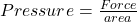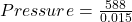## A person weighs 60 kg. The area under the foot of the person is 150 cm2. Find the pressure exerted on the ground by the person.

Question

A person weighs 60 kg. The area under the foot of the person is 150 cm2. Find the pressure exerted on the ground by the person.

in progress 0
2 months 2021-07-25T16:36:31+00:00 1 Answers 5 views 0

Pressure = 39200 N/m²

Explanation:

Given the following data;

Mass = 60 kg

Area = 150 cm²

Conversion:

1 centimeter square = 0.0001 meter square

150 centimeter square = 150 * 0.0001 = 0.015 m²

To find the pressure exerted on the ground by the person;

Mathematically, pressure is given by the formula;First of all, we would determine the force exerted by the person.

Force = mass * acceleration due to gravity.

We know that acceleration due to gravity is equal to 9.8 m/s².

Substituting into the formula, we have;

Force = 60 * 9.8

Force = 588 Newton

Next, find the pressure;Substituting into the formula, we have;Pressure = 39200 N/m²